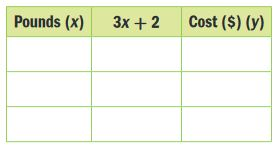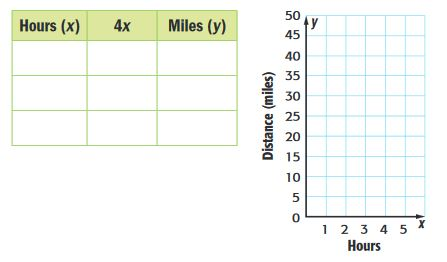Homework Explained - Math Practice 101Dear guest, you are not a registered member. As a guest, you only have read-only access to our books, tests and other practice materials.

As a registered member you can:

Registration is free and doesn't require any type of payment information. Click here to Register.
Go to page:
Chapter 8:Functions and Inequalities;Lesson 1:Function Tables

### Guided Practice

• Question 1
Isaiah is buying jelly beans. In bulk, they cost $3 per pound, and a candy dish costs$2. The function rule, 3x + 2 where x is the number of pounds, can be used to find the total cost of x pounds of jelly beans and 1 dish. Make a table that shows the total cost of buying 2, 3, or 4 pounds of jelly beans and 1 dish.• Question 2

Jasper hikes 4 miles per hour. The function rule that represents this situation is 4x, where x is the number of hours. Make a table to find how many hours he has hiked when he has gone 8, 12, and 20 miles. Then graph the function.• Question 3
Building on the Essential Question How can a function table help you find input or output?

Yes, email page to my online tutor. (if you didn't add a tutor yet, you can add one here)